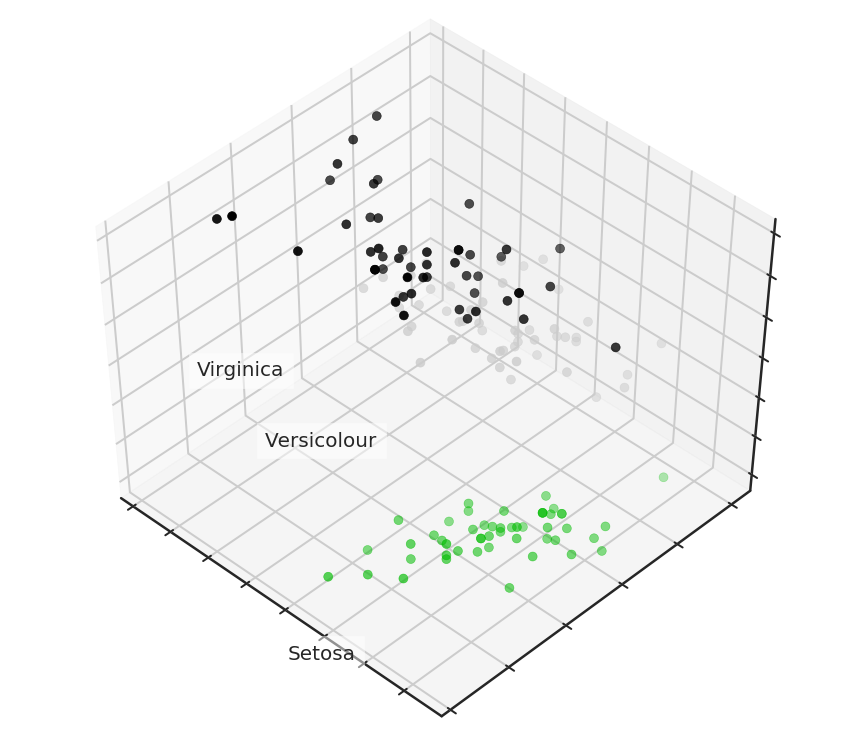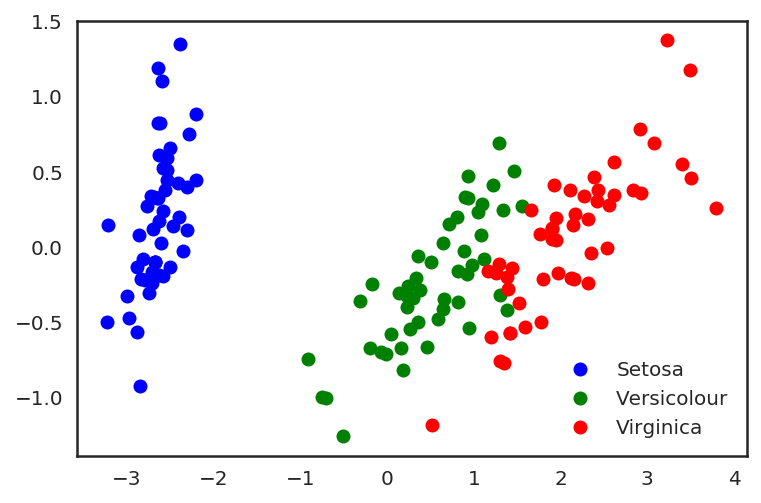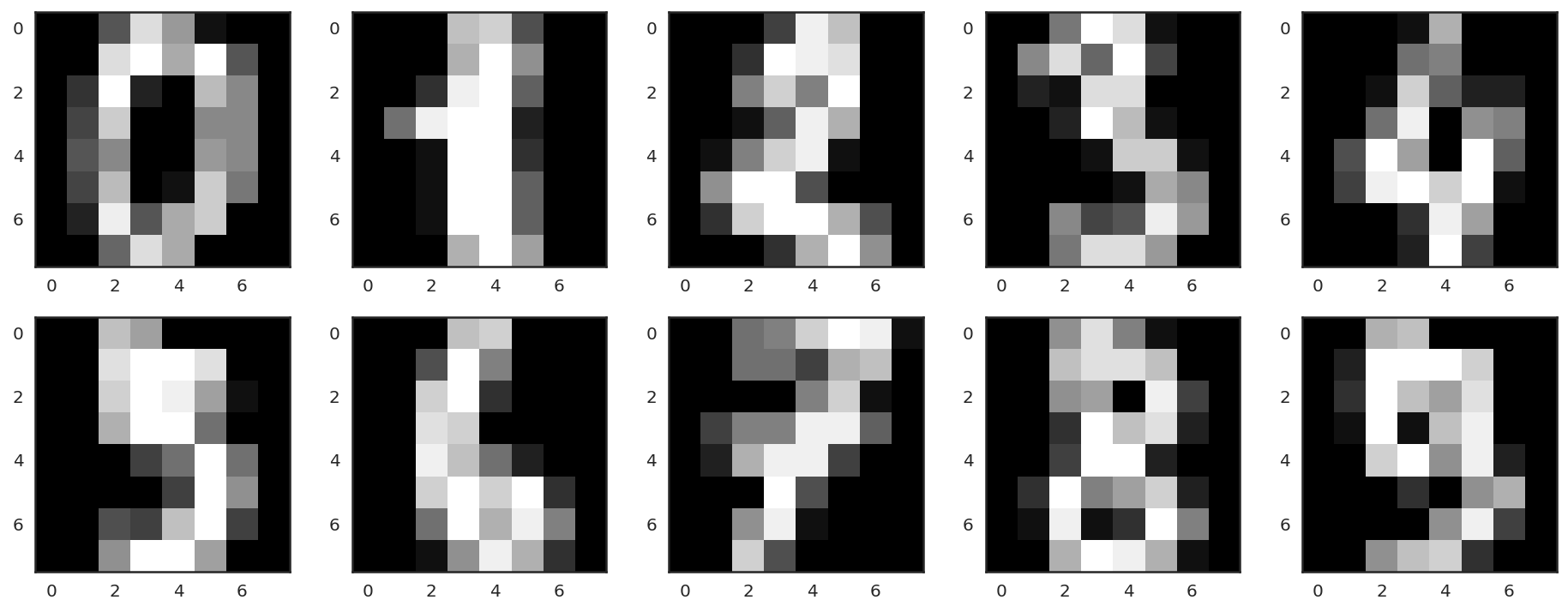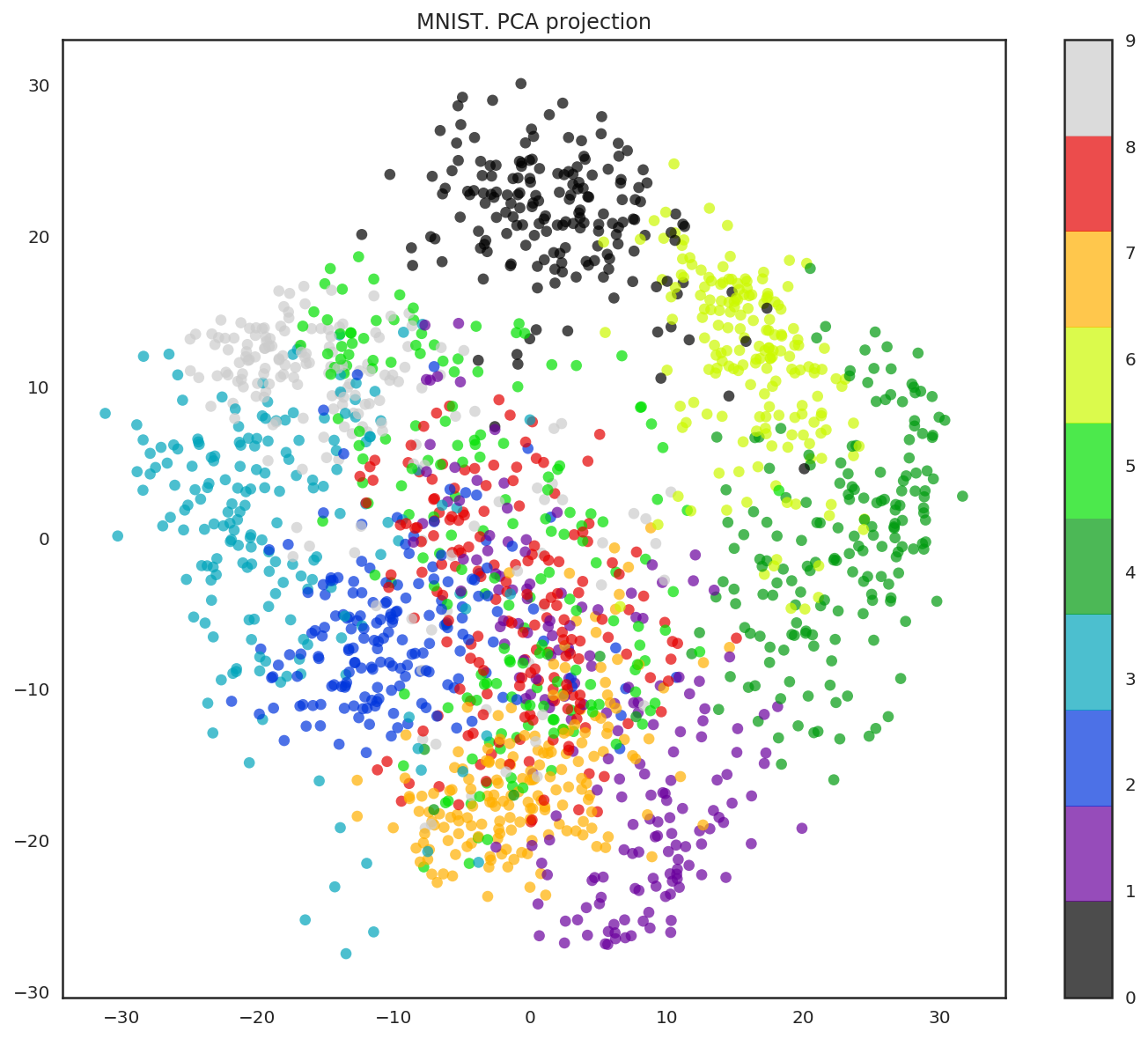## Open Machine Learning Course¶

Author: Sergey Korolev, Software Engineer at Snap Inc.
Translated and edited by Egor Polusmak, Anastasia Manokhina, Anna Golovchenko, Eugene Mashkin, and Yuanyuan Pao.

This material is subject to the terms and conditions of the license Creative Commons CC BY-NC-SA 4.0. Free use is permitted for any non-comercial purpose with an obligatory indication of the names of the authors and of the source.

# Topic 7. Unsupervised learning: PCA and clustering

Welcome to the seventh part of our Open Machine Learning Course!

In this lesson, we will work with unsupervised learning methods such as Principal Component Analysis (PCA) and clustering. You will learn why and how we can reduce the dimensionality of the original data and what the main approaches are for grouping similar data points.

### Article outline¶

1. Introduction
2. PCA
• Intuition, theories, and application issues
• Application examples
3. Cluster analysis
• K-means
• Affinity Propagation
• Spectral clustering
• Agglomerative clustering
• Accuracy metrics

## 1. Introduction¶

The main feature of unsupervised learning algorithms, when compared to classification and regression methods, is that input data are unlabeled (i.e. no labels or classes given) and that the algorithm learns the structure of the data without any assistance. This creates two main differences. First, it allows us to process large amounts of data because the data does not need to be manually labeled. Second, it is difficult to evaluate the quality of an unsupervised algorithm due to the absence of an explicit goodness metric as used in supervised learning.

One of the most common tasks in unsupervised learning is dimensionality reduction. On one hand, dimensionality reduction may help with data visualization (e.g. t-SNE method) while, on the other hand, it may help deal with the multicollinearity of your data and prepare the data for a supervised learning method (e.g. decision trees).

## 2. Principal Component Analysis (PCA)¶

### Intuition, theories, and application issues¶

Principal Component Analysis is one of the easiest, most intuitive, and most frequently used methods for dimensionality reduction, projecting data onto its orthogonal feature subspace.More generally speaking, all observations can be considered as an ellipsoid in a subspace of an initial feature space, and the new basis set in this subspace is aligned with the ellipsoid axes. This assumption lets us remove highly correlated features since basis set vectors are orthogonal. In the general case, the resulting ellipsoid dimensionality matches the initial space dimensionality, but the assumption that our data lies in a subspace with a smaller dimension allows us to cut off the "excessive" space with the new projection (subspace). We accomplish this in a 'greedy' fashion, sequentially selecting each of the ellipsoid axes by identifying where the dispersion is maximal.

"To deal with hyper-planes in a 14 dimensional space, visualize a 3D space and say 'fourteen' very loudly. Everyone does it." - Geoffrey Hinton

Let's take a look at the mathematical formulation of this process:

In order to decrease the dimensionality of our data from $n$ to $k$ with $k \leq n$, we sort our list of axes in order of decreasing dispersion and take the top-$k$ of them.

We begin by computing the dispersion and the covariance of the initial features. This is usually done with the covariance matrix. According to the covariance definition, the covariance of two features is computed as follows: $$cov(X_i, X_j) = E[(X_i - \mu_i) (X_j - \mu_j)] = E[X_i X_j] - \mu_i \mu_j,$$ where $\mu_i$ is the expected value of the $i$th feature. It is worth noting that the covariance is symmetric, and the covariance of a vector with itself is equal to its dispersion.

Therefore the covariance matrix is symmetric with the dispersion of the corresponding features on the diagonal. Non-diagonal values are the covariances of the corresponding pair of features. In terms of matrices where $\mathbf{X}$ is the matrix of observations, the covariance matrix is as follows:

$$\Sigma = E[(\mathbf{X} - E[\mathbf{X}]) (\mathbf{X} - E[\mathbf{X}])^{T}]$$

Quick recap: matrices, as linear operators, have eigenvalues and eigenvectors. They are very convenient because they describe parts of our space that do not rotate and only stretch when we apply linear operators on them; eigenvectors remain in the same direction but are stretched by a corresponding eigenvalue. Formally, a matrix $M$ with eigenvector $w_i$ and eigenvalue $\lambda_i$ satisfy this equation: $M w_i = \lambda_i w_i$.

The covariance matrix for a sample $\mathbf{X}$ can be written as a product of $\mathbf{X}^{T} \mathbf{X}$. According to the Rayleigh quotient, the maximum variation of our sample lies along the eigenvector of this matrix and is consistent with the maximum eigenvalue. Therefore, the principal components we aim to retain from the data are just the eigenvectors corresponding to the top-$k$ largest eigenvalues of the matrix.

The next steps are easier to digest. We multiply the matrix of our data $X$ by these components to get the projection of our data onto the orthogonal basis of the chosen components. If the number of components was smaller than the initial space dimensionality, remember that we will lose some information upon applying this transformation.

## Examples¶

### Fisher's iris dataset¶

Let's start by uploading all of the essential modules and try out the iris example from the scikit-learn documentation.

In :
import numpy as np
import matplotlib.pyplot as plt
import seaborn as sns; sns.set(style='white')
%matplotlib inline
%config InlineBackend.figure_format = 'retina'
from sklearn import decomposition
from sklearn import datasets
from mpl_toolkits.mplot3d import Axes3D

X = iris.data
y = iris.target

# Let's create a beautiful 3d-plot
fig = plt.figure(1, figsize=(6, 5))
plt.clf()
ax = Axes3D(fig, rect=[0, 0, .95, 1], elev=48, azim=134)

plt.cla()

for name, label in [('Setosa', 0), ('Versicolour', 1), ('Virginica', 2)]:
ax.text3D(X[y == label, 0].mean(),
X[y == label, 1].mean() + 1.5,
X[y == label, 2].mean(), name,
horizontalalignment='center',
bbox=dict(alpha=.5, edgecolor='w', facecolor='w'))
# Change the order of labels, so that they match
y_clr = np.choose(y, [1, 2, 0]).astype(np.float)
ax.scatter(X[:, 0], X[:, 1], X[:, 2], c=y_clr,
cmap=plt.cm.nipy_spectral)

ax.w_xaxis.set_ticklabels([])
ax.w_yaxis.set_ticklabels([])
ax.w_zaxis.set_ticklabels([]);Now let's see how PCA will improve the results of a simple model that is not able to correctly fit all of the training data:

In :
from sklearn.tree import DecisionTreeClassifier
from sklearn.model_selection import train_test_split
from sklearn.metrics import accuracy_score, roc_auc_score

# Train, test splits
X_train, X_test, y_train, y_test = train_test_split(X, y, test_size=.3,
stratify=y,
random_state=42)

# Decision trees with depth = 2
clf = DecisionTreeClassifier(max_depth=2, random_state=42)
clf.fit(X_train, y_train)
preds = clf.predict_proba(X_test)
print('Accuracy: {:.5f}'.format(accuracy_score(y_test,
preds.argmax(axis=1))))

Accuracy: 0.88889


Let's try this again, but, this time, let's reduce the dimensionality to 2 dimensions:

In :
# Using PCA from sklearn PCA
pca = decomposition.PCA(n_components=2)
X_centered = X - X.mean(axis=0)
pca.fit(X_centered)
X_pca = pca.transform(X_centered)

# Plotting the results of PCA
plt.plot(X_pca[y == 0, 0], X_pca[y == 0, 1], 'bo', label='Setosa')
plt.plot(X_pca[y == 1, 0], X_pca[y == 1, 1], 'go', label='Versicolour')
plt.plot(X_pca[y == 2, 0], X_pca[y == 2, 1], 'ro', label='Virginica')
plt.legend(loc=0);In :
# Test-train split and apply PCA
X_train, X_test, y_train, y_test = train_test_split(X_pca, y, test_size=.3,
stratify=y,
random_state=42)

clf = DecisionTreeClassifier(max_depth=2, random_state=42)
clf.fit(X_train, y_train)
preds = clf.predict_proba(X_test)
print('Accuracy: {:.5f}'.format(accuracy_score(y_test,
preds.argmax(axis=1))))

Accuracy: 0.91111


The accuracy did not increase significantly in this case, but, with other datasets with a high number of dimensions, PCA can drastically improve the accuracy of decision trees and other ensemble methods.

Now let's check out the percent of variance that can be explained by each of the selected components.

In :
for i, component in enumerate(pca.components_):
print("{} component: {}% of initial variance".format(i + 1,
round(100 * pca.explained_variance_ratio_[i], 2)))
print(" + ".join("%.3f x %s" % (value, name)
for value, name in zip(component,
iris.feature_names)))

1 component: 92.46% of initial variance
0.362 x sepal length (cm) + -0.082 x sepal width (cm) + 0.857 x petal length (cm) + 0.359 x petal width (cm)
2 component: 5.3% of initial variance
0.657 x sepal length (cm) + 0.730 x sepal width (cm) + -0.176 x petal length (cm) + -0.075 x petal width (cm)


### Handwritten numbers dataset¶

Let's look at the handwritten numbers dataset that we used before in the 3rd lesson.

In :
digits = datasets.load_digits()
X = digits.data
y = digits.target


Let's start by visualizing our data. Fetch the first 10 numbers. The numbers are represented by 8 x 8 matrixes with the color intensity for each pixel. Every matrix is flattened into a vector of 64 numbers, so we get the feature version of the data.

In :
# f, axes = plt.subplots(5, 2, sharey=True, figsize=(16,6))
plt.figure(figsize=(16, 6))
for i in range(10):
plt.subplot(2, 5, i + 1)
plt.imshow(X[i,:].reshape([8,8]), cmap='gray');Our data has 64 dimensions, but we are going to reduce it to only 2 and see that, even with just 2 dimensions, we can clearly see that digits separate into clusters.

In :
pca = decomposition.PCA(n_components=2)
X_reduced = pca.fit_transform(X)

print('Projecting %d-dimensional data to 2D' % X.shape)

plt.figure(figsize=(12,10))
plt.scatter(X_reduced[:, 0], X_reduced[:, 1], c=y,
edgecolor='none', alpha=0.7, s=40,
cmap=plt.cm.get_cmap('nipy_spectral', 10))
plt.colorbar()
plt.title('MNIST. PCA projection');

Projecting 64-dimensional data to 2DIndeed, with t-SNE, the picture looks better since PCA has a linear constraint while t-SNE does not. However, even with such a small dataset, the t-SNE algorithm takes significantly more time to complete than PCA.

In :
%%time

from sklearn.manifold import TSNE
tsne = TSNE(random_state=17)

X_tsne = tsne.fit_transform(X)

plt.figure(figsize=(12,10))
plt.scatter(X_tsne[:, 0], X_tsne[:, 1], c=y,
edgecolor='none', alpha=0.7, s=40,
cmap=plt.cm.get_cmap('nipy_spectral', 10))
plt.colorbar()
plt.title('MNIST. t-SNE projection');

CPU times: user 25.4 s, sys: 2.94 s, total: 28.4 s
Wall time: 28.4 s